This is a quick introduction to the OpenSky data set for the aircraft localization competition. The goal of the competition is to find the locations of some aircraft based on time-difference of arrival and signal strength measurements provided by a network of sensors on the ground.

# The Data Set

## Measurements

Let’s begin with the data set.

library(data.table)

measurements = fread("training_1_category_4.csv")

The measurements CSV files are structured like this:

# show two random lines
rndi = sample(1:nrow(measurements), 2)
knitr::kable(measurements[rndi], format="html", format.args=list(full_width=T))
id timeAtServer aircraft latitude longitude baroAltitude geoAltitude numMeasurements measurements
1439066 929.714 179 46.55903 14.060371 11277.60 11506.20 2 [[114,930672432312,116],[131,930672970687,74]]
2446659 1560.153 1640 42.95801 4.661205 10660.38 10904.22 2 [[132,1561125919656,56],[432,1566991243166.67,65]]

### Column descriptions

• id: a unique ID for each transponder transmission. This ID can be used to refer to specific measurements in the results file.
• timeAtServer: timestamp denoting the time when the measurement arrived at OpenSky’s server. Unit is seconds and it starts at timeAtServer=0 in each data set.
• aircraft: a randomized ID of the aircraft which sent the position report.
• latitude: latitude reported by the aircraft in decimal degrees. This column is null for those positions which should be determined by the localization algorithm.
• longitude: longitude reported by the aircraft in decimal degrees. This column is null for those positions which should be determined by the localization algorithm.
• baroAltitude: barometric altitude reported by the aircraft in meters.
• geoAltitude: geometric (GPS) height reported by the aircraft in meters. This column is null for those positions which should be determined by the localization algorithm.
• numMeasurements: redundant field indicating the number of sensors which recorded the position report.
• measurements: JSON array of triples [sensorID, timestamp, signalstrength].
• serial: unique sensor ID which can be matched with the sensor information table (sensors data table below).
• timestamp: precise timestamp for the detection of the position report at the sensor in nanoseconds.
• signalstrength: indicator of the strength of the report’s signal at the sensor (often in dB).

## Sensor information

You will also need additional information about the sensors during the competition.

# load the sensor information
sensors = fread("sensors.csv")

How is the sensor information structured?

# show two random lines
rndi = sample(1:nrow(sensors), 2)
knitr::kable(sensors[rndi], format="html", format.args=list(full_width=T))
serial latitude longitude height type
178 58.24373 -6.351554 20 dump1090
417 47.37750 8.236600 417 dump1090

### Column descriptions

• serial: unique sensor ID which can be used to join the sensor information with the measurements data.
• latitude: latitude of the sensor in decimal degrees. It has been reported either by the sensor hardware or manually by the sensor operator.
• longitude: longitude of the sensor in decimal degrees. It has been reported either by the sensor hardware or manually by the sensor operator.
• height: height of the sensor in meters. Is has been reported either by the sensor hardware or manually by the sensor operator.
• type: type of the sensor hardware that was used to record the measurements.

# Example Flight

As mentioned above, you will have to look at the timestamps (or rather the differences of timestamps) and the signal strength measurements to find the locations of the unknown aircraft. So let’s have a look at the measurement data for the specific flight of aircraft number 6 to see how it all fits together.

I will start by plotting a map with the flight an the sensor positions.

# select the data of aircraft number 1716
flight = measurements[aircraft==1716]

# I prefer ggplot2 and ggmap for plotting stuff on maps
library(ggplot2)
library(ggmap)

world <- data.table(map_data("world"))
names(world)[names(world)=="lat"] <- "latitude"
names(world)[names(world)=="long"] <- "longitude"

# the map limits
xlims = range(flight$longitude) ylims = range(flight$latitude)

# plot the map
ggplot(flight, aes(x=longitude, y=latitude)) +
geom_map(data=world, map=world, aes(map_id=region),
color="gray", fill="#e3e3e3", size=0.1) +
geom_point(aes(color=geoAltitude), size=1) +
geom_point(data=sensors, color="coral", size=1) +
xlim(xlims-1, xlims+1) + ylim(ylims-1, ylims+1) +
theme(axis.line = element_blank(), axis.text = element_blank(),
axis.title = element_blank(), axis.ticks = element_blank(),
panel.background = element_blank())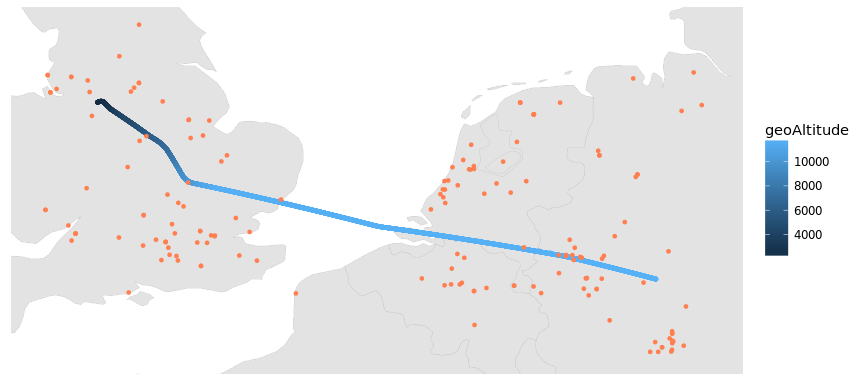Interesting! So apparently this flight was over Germany at the beginning of the data set and was just about to land at Manchester airport when the recording stopped an hour later. The red dots are the positions of the sensors that are located nearby the flight.

So how does the measurement data of this flight actually look like?

## Timestamp measurements

# extract the measurement data from the JSON array
library(jsonlite)
flattened = rbindlist(lapply(flight$measurements, function (m) data.table(fromJSON(m))), idcol=T) colnames(flattened) = c("i", "serial", "timestamp", "signalStrength") # join the two data sets to get the positions flight$i = 1:nrow(flight)
flattened = merge(flattened,
flight[, -c("measurements", "numMeasurements")],
by="i")

# plot the timestamps over time (one color for each sensor)
ggplot(flattened, aes(x=timeAtServer, y=timestamp,
color=factor(serial))) +
geom_point(size=0.1) + guides(color = "none") +
xlab("Observation Time (s)") + ylab("Timestamp (ns)")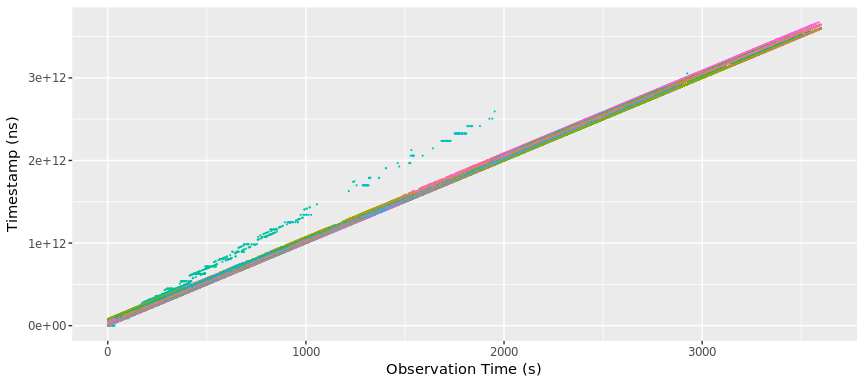It’s not very surprising that the nanosecond timestamp counts from about 0 to about 3600e9 over a duration of 3600 seconds. However, this plot already shows some of the problems we’ll face during the competition: the timestamps of some sensors are broken (here: green)! Maybe the difference between timestamps of different sensors tells us something about the location of the aircraft?

# calculate the set of timestamp differences for a given position report
combineMeasurements <- function(s, m) {
# always use the smaller serial as "left" sensor to
# have a globally unique order within each pair
sorted = order(s)
idc = combn(sorted, 2)
return(data.table(
serial1 = s[idc[1,]], serial2 = s[idc[2,]],
delta = m[idc[1,]] - m[idc[2,]]
))
}

# generate the timestamp differences for aircraft #6
tsdelta = flattened[, combineMeasurements(serial, timestamp), by=i]

tsdelta = merge(tsdelta, flight[, .(i, timeAtServer)], by="i")

# and plot the timestamp differences (again, one color for each pair of sensors)
ggplot(tsdelta, aes(x=timeAtServer, y=delta,
color=sprintf("%d/%d", serial1, serial2))) +
geom_point(size=0.1) + guides(color = "none") +
xlab("Observation Time (s)") + ylab("Timestamp Difference (ns)")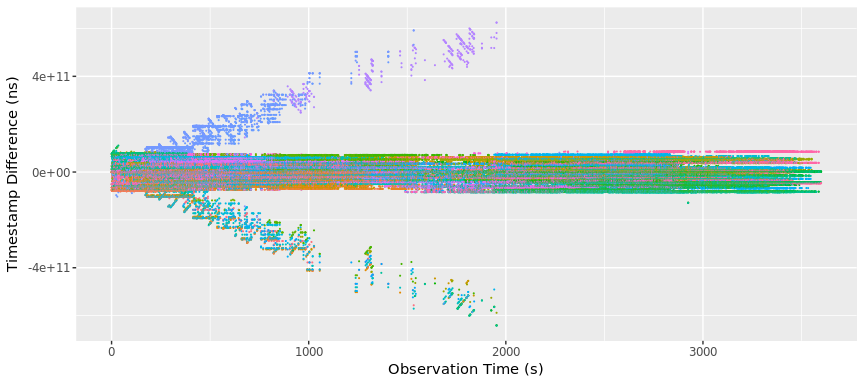Interesting, there are patterns! The sensor with the scrambled timestamps leads to those two branches leading away from the abscissa when combined with the timestamps of other sensors. Let’s have a look at the timestamp differences of a “good” pair of sensors:

s1 = 168
s2 = 191

# select the data for the pair
mask = tsdelta$serial1==s1 & tsdelta$serial2==s2

# and plot them (one color for each pair of sensors)
geom_point(size=0.1) + guides(color = "none") +
xlab("Observation time (s)") + ylab("Timestamp Difference (ns)")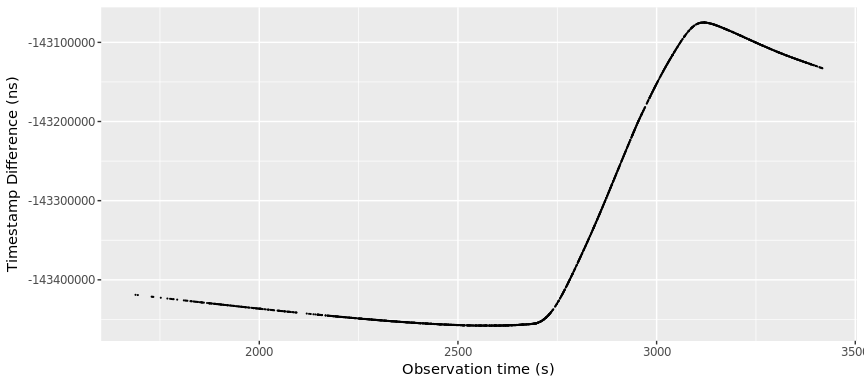That looks much more interesting! Something seems to happen here. Spoiler alert: the time difference correlates with the distance difference! Let’s check this.

# add aircraft location information to the table
tsdelta = merge(tsdelta, flight[, .(i, latitude, longitude, geoAltitude)], by="i")
setnames(tsdelta, "geoAltitude", "height")

# calculate the distances from the sensor to the aircraft
tsdelta$dist1 = dist3d(tsdelta, sensors[s1]) tsdelta$dist2 = dist3d(tsdelta, sensors[s2])

# and plot the distance differences
geom_point(size=0.1) + guides(color = "none") +
xlab("Observation time (s)") + ylab("Distance Difference (km)")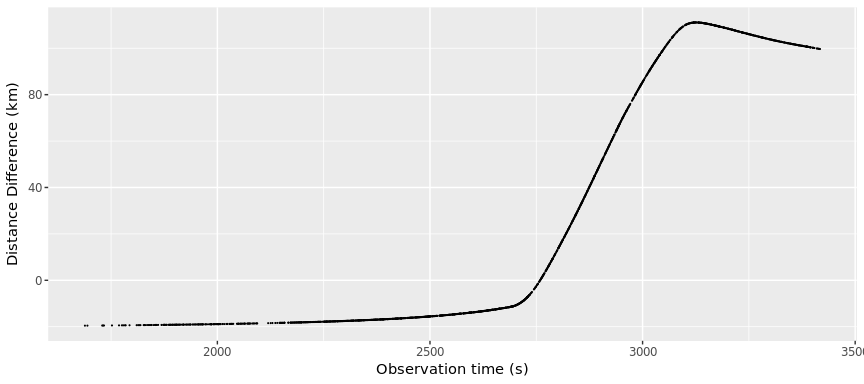Huh, what a coincidence! So in the beginning, the aircraft was slightly closer to sensor 168. At about t=2750 seconds, the aircraft turned towards sensor 191 and distance to sensor 168 exceeded that to sensor 191. As a result, the difference became positive. How does this look like on a map?

# the map limits
xlims = range(c(tsdelta$longitude[mask], sensors$longitude[c(s1, s2)]))
ylims = range(c(tsdelta$latitude[mask], sensors$latitude[c(s1, s2)]))

geom_map(data=world, map=world, aes(map_id=region),
color="gray", fill="#e3e3e3", size=0.1) +
geom_point(aes(color=height), size=1) +
geom_point(data=sensors[c(s1,s2)], color="coral", size=1) +
xlim(xlims-1, xlims+1) + ylim(ylims-1, ylims+1) +
theme(axis.line = element_blank(), axis.text = element_blank(),
axis.title = element_blank(), axis.ticks = element_blank(),
panel.background = element_blank())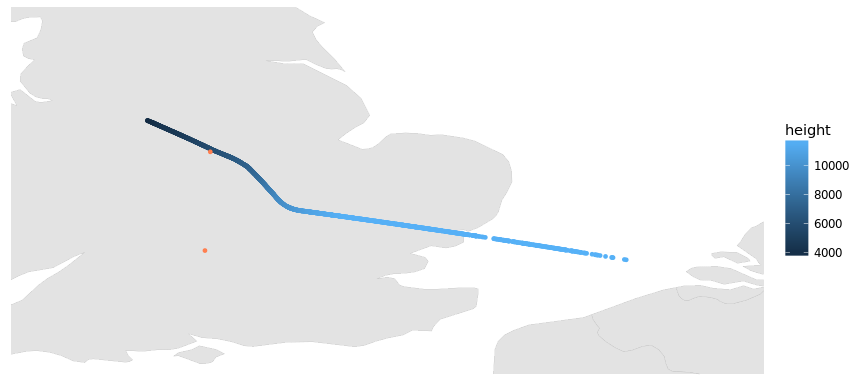## Signal strength measurements

The signal strength values of all sensors which recorded position reports of aircraft number 6 are:

# a different color for each sensor
ggplot(flattened, aes(x=timeAtServer, y=signalStrength,
color=factor(serial))) +
geom_point(size=0.1) + guides(color = "none") +
xlab("Observation Time (s)") + ylab("Signal Strength (dB)")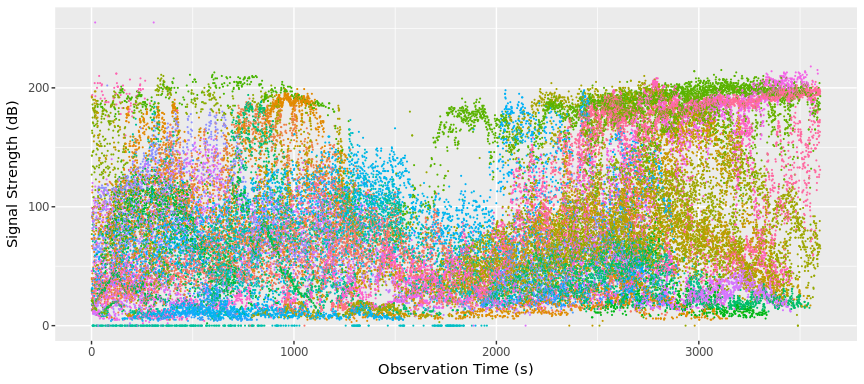Nice, modern art! And there are clear patterns visible. Spoiler alert: the signal strength correlates with the distance (and many other things). Let’s have a look at the signal strength measurements from one of the sensors of our favorit pair:

# select the data of one sensor
signalstrengths = flattened[serial==sensors$serial[s1]] setnames(signalstrengths, "geoAltitude", "height") signalstrengths$dist = dist3d(signalstrengths, sensors[s1])

# create a stacked plot with the distance and the signal strength
p1 <- ggplot(signalstrengths, aes(x=unixtime(timeAtServer))) +
geom_point(aes(y=signalStrength), size=0.1) +
xlab(NULL) + ylab("Signal Strength (dB)") +
guides(color = "none")

p2 <- ggplot(signalstrengths, aes(x=unixtime(timeAtServer))) +
geom_point(aes(y=dist/1e3), size=0.1) +
xlab("Observation Time (s)") + ylab("Distance (km)") +
guides(color = "none")

library(gtable)
library(grid) # low-level grid functions are required
g1 <- ggplotGrob(p1)
g2 <- ggplotGrob(p2)
g <- rbind(g1, g2, size="first") # stack the plots
g$widths <- unit.pmax(g1$widths, g2$widths) # use the largest widths # center the legend vertically #g$layout[grepl("guide", g$layout$name),c("t","b")] <- c(1,nrow(g))
grid.newpage()
grid.draw(g)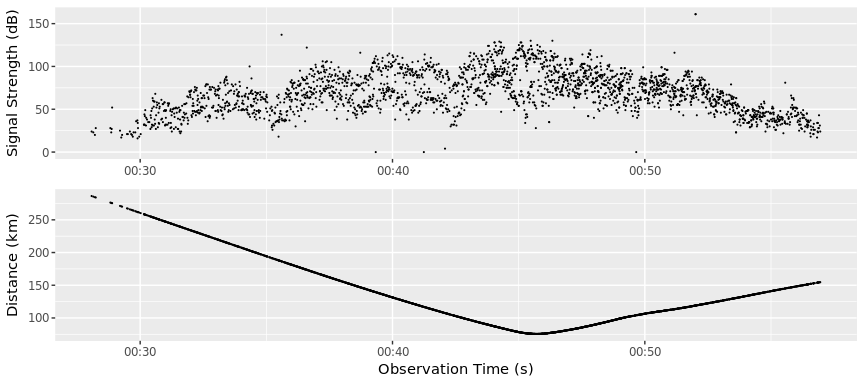Much noiser but the correlation is clearly visible!

# Conclusion

So it seems that the measurement data can be used to infer information on the aircraft positions. But beware: the above example was cherry picking! There is much noise in the data, people might lie about the exact locations of their sensors, most clocks will drift, and many other problems are looming on the horizon. You might have to ask the other aircraft for help!

Can you find the missing aircraft positions?

# Appendix (Helper Functions)

Here’s the code of some of the little helpers I used above.

## WSG84 Coordinates

# convert decimal degrees to radians
degrees*pi/180

# convert radians to decimal degrees

# convert WSG84 coordinates to cartesian ones
lla2ecef <- function(lla) {
lat = rad(lla$latitude) lon = rad(lla$longitude)

# WSG84 ellipsoid constants
a = 6378137
e = 8.1819190842622e-2

# prime vertical radius of curvature
N = a / sqrt(1 - e^2 * sin(lat)^2)

return(data.table(
x = (N+lla$height) * cos(lat) * cos(lon), y = (N+lla$height) * cos(lat) * sin(lon),
z = ((1-e^2) * N + lla$height) * sin(lat) )) } # Euclidean distance euclideanDist <- function(c1, c2) return(sqrt((c1$x-c2$x)^2 + (c1$y-c2$y)^2 + (c1$z-c2\$z)^2))

# 3D distance between WSG84 coordinates
dist3d <- function(lla1, lla2)
euclideanDist(lla2ecef(lla1), lla2ecef(lla2))